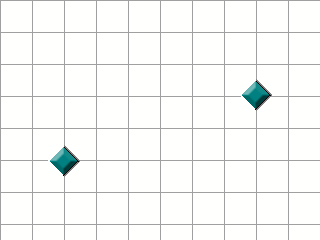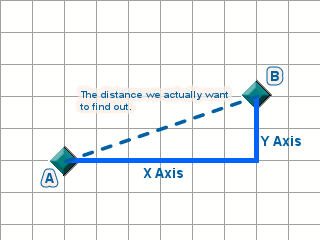1. ## Distance Formula

[color:#0088aa][size:17pt]Distance Formula[/size][/color]
[color:#0088aa]How Distance Formula Works[/color]

[color:#000099]

The purpose of this article is to explain how to work out the distance between two points. Some maths is involved, but I'll try and explain it as simply as possible (I've got dyscalculia, of which fact I am immensely proud, so don't go saying you're bad at maths and therefore can't do it.).

So anyway, it really is pretty straightforward, so let's start.

[size:14pt][color:#0088aa]Our Objects[/color][/size]

Here are our two objects. We're gonna find the distance between them:

&nbsp;&nbsp;&nbsp;&nbsp;&nbsp;&nbsp;&nbsp;&nbsp;&n bsp;&nbsp;&nbsp;&nbsp;&nbsp;&nbsp;&nbsp;&nbsp;&nbs p;&nbsp;First bit is pretty straightforward, I'm assuming you get the stuff about X and Y axes (x goes along the top, y goes down the left).

So if we count how far apart they are on the X and Y axis, we get this:

X distance = 6 squares apart
Y distance = 2 squares apart

We can do that with the following MMF expressions:

Code:
```x("Object A") - x("Object B") //The X Distance

y("Object A") - y("Object B") //The Y Distance```
Simply put, we're taking the coordinates of one object, and subtracting them from another. There will obviously be a difference left over between the two, and that is our distance.

So far so good, but this gives us two distances. One for the X axis, one for the Y axis. We need to combine them into one single value.

To do that, we need to move on and see how - funnily enough - this whole thing has a lot to do with Triangles.

[size:14pt][color:#0088aa]Triangles, You Say?[/color][/size]

Oui, monsieur. Sim, senhor. Ja, mein herr. Etc.

Look at our diagram of the two objects now. For simplicity, I've named them A and B as well, so we can tell them apart:

&nbsp;&nbsp;&nbsp;&nbsp;&nbsp;&nbsp;&nbsp;&nbsp;&n bsp;&nbsp;&nbsp;&nbsp;&nbsp;&nbsp;&nbsp;&nbsp;&nbs p;&nbsp;We know the X and Y distances, we can find them easily as I showed above, by taking the coordinates of the one object and subtracting it from the other. The remaining amount is the distance between the two on that axis.

But now, we must combine them. How? Well do you notice how we're forming a kind of triangle? The top (dotted) line is the only line whose length we don't already know.

How handy it is, then, that about 2580 years ago, a Greek chap called Pythagoras found out just how to deal with our very problem.[size:14pt][color:#0088aa]What The Greek Dude Said About Triangles[/color][/size]

Pythagoras found out that, in a right-angled triangle (the kind we're dealing with here), the angled line (called the Hypotenuse) has a very basic relationship to the two straight lines.

To explain it better, look at this diagram from Wikipedia:

&nbsp;&nbsp;&nbsp;&nbsp;&nbsp;&nbsp;&nbsp;&nbsp;&n bsp;&nbsp;&nbsp;&nbsp;&nbsp;&nbsp;&nbsp;&nbsp;&nbs p;&nbsp;&nbsp;&nbsp;&nbsp;&nbsp;&nbsp;&nbsp;&nbsp; &nbsp;&nbsp;&nbsp;&nbsp;&nbsp;&nbsp;&nbsp;&nbsp;&n bsp;&nbsp;&nbsp;On this diagram, a is the Y Distance, and b is the X Distance. c is the total distance, which we want to find out.

Pythagoras takes the X Distance, and the Y Distance, and he uses them to create a couple of squares (the straight a and b squares you see on the diagram).

He then adds them together, creating a kind of Super Square (a bigger square, basically).

That Super Square will look very much like square c, and the length of each one of its sides will be... the length of the distance that we are looking for.

Confused? It will become simplerWe'll do a worked example.

[size:14pt][color:#0088aa]Worked Example[/color][/size]

So, we're gonna work through the example we mentioned at the outset, and then there'll be a nice example file for you to look through at your leisure.&nbsp;&nbsp;&nbsp;&nbsp;&nbsp;&nbsp;&nbsp;&nbsp;&n bsp;&nbsp;&nbsp;&nbsp;&nbsp;&nbsp;&nbsp;&nbsp;&nbs p;&nbsp;STEP ONE

Okay, so as we did above, count how many rows (Y) and columns (the two objects are apart in our diagram. We'll imagine each grid square on the diagram is a pixel.

You'll find they are:

X distance = 6 pixels apart
Y distance = 2 pixels apart

In MMF, we do this:
Code:
```X Distance = x("Object A") - x("Object B")
Y Distance = y("Object A") - y("Object B")```
STEP TWO
&nbsp;&nbsp;&nbsp;&nbsp;&nbsp;&nbsp;&nbsp;&nbsp;&n bsp;&nbsp;&nbsp;&nbsp;&nbsp;&nbsp;&nbsp;&nbsp;&nbs p;&nbsp;&nbsp;&nbsp;&nbsp;&nbsp;&nbsp;&nbsp;&nbsp; &nbsp;&nbsp;&nbsp;&nbsp;&nbsp;&nbsp;&nbsp;&nbsp;&n bsp;&nbsp;&nbsp;Turn those distances into squares. You can do this in Windows Calculator by typing in the distances and squaring them. The result:

X distance = 6² = 36 pixels
Y distance = 2² = 4 pixels

...so now we have the area of two squares, right?

In MMF, there is no square button, but you can type 'POW 2' to get the same effect. So:

Code:
```X square = X Distance POW 2
Y square = Y Distance POW 2```

STEP THREE

Big Square = 36 + 4 = 40 pixels

So now we have the area of our even-bigger-square, our Super Square.

I'm sure I don't need to tell you that in MMF it would be:

Code:
`Big Square = X Square + Y Square`

STEP FOUR

Now that we have our Big Square, we need to find out how long its sides are. So bring up your Windows Calculator, and use the Square Root function. This is like flattening the square.

Flattened Big Square = √40 = 6.3245 pixels

And that's the total distance!!In MMF that's:
Code:
`Total Distance = SQR(Big Square)`
And that's it!!

The Final Formula Becomes...

Code:
```sqr(
( x("Object A") - x("Object B") ) POW 2
+ ( y("Object A") - y("Object B") ) POW 2
)```
Heh... That may well have confused you, but even if it has, the basis is there in your head now.

Look in the attached example, and hopefully seeing it in practical use will make things a little better. It also comes with examples of cool ways you can use this formula.You can of course do all of this using the Direction Calculator Object, but it can be useful to know how to do it without.

I hope this helped some, any comments on how I can improve this article are appreciated[/color]2. ## Re: Distance Formula

Yet another great article from Dines. The only problem I have with it is the X and Y distances part. You have:

X Distance = x("Object A") - x("Object B")
Y Distance = y("Object A") - y("Object B")

but shouldn't it be:

X Distance = abs(x("Object A") - x("Object B"))
Y Distance = abs(y("Object A") - y("Object B"))

Just so you don't end up with negative values incase object B has a larger position value than object A.3. ## Re: Distance Formula

Yep Brandon is probably right. It should be absolute values.

I can't understand how you can write a such big article about this.4. ## Re: Distance Formula

Lol, yeah, oops :blush:5. ## Re: Distance Formula

Abs() is not needed, when you multiply something with itself the answer is always positive. -2 * -2 = 46. ## Re: Distance Formula

LoL that's true! xD7. ## Re: Distance Formula

You are a genius in math(s)8. ## Re: Distance FormulaOriginally Posted by Werbad
Abs() is not needed, when you multiply something with itself the answer is always positive. -2 * -2 = 4
What do you mean? I'm not talking about the actual distance formula, of course you don't need to use absolute values for that. I'm talking about earlier on how Dines mentions how to find X and Y distances separately, which does require an absolute value to make it positive in some cases, because after all you can't have a negative distance. I think we are talking about two different things because there is no multiplying in what I was referring to, only simple subtraction.9. ## Re: Distance Formula

We just learnt Pythagoras theorem in year 8 mathsGreat job Dines!10. ## Re: Distance Formula

Just making sure nobody would add Abs() to the Distance formula thinking there was an error in Dines article...####Posting Permissions

• You may not post new threads
• You may not post replies
• You may not post attachments
• You may not edit your posts
•